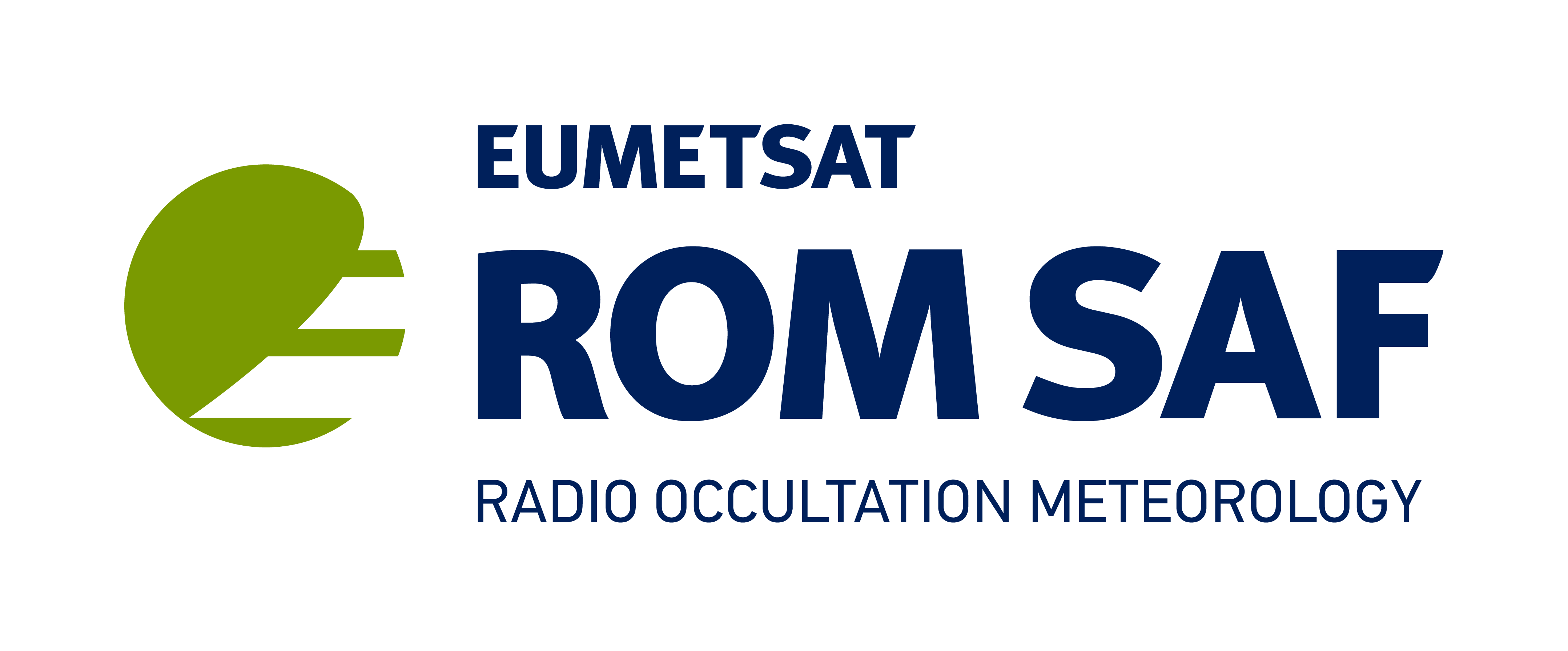# GPSRO monitoringThe GPS radio occultation (GPSRO) is a limb measurement that is based on Snells law of refraction. The path of a radio signal propagating between a GPS satellite and a receiver on a low earth orbiting (LEO) satellite is bent as a result of refractive index gradients in the atmosphere. The bending angle contains useful information on the atmospheric temperature and humidity.

The technique has good vertical resolution and an all-weather capability. GPSRO provides particularly accurate, sub-Kelvin temperature information in the upper-troposphere and lower-stratosphere. GPSRO measurements are currently available from METOP-A/GRAS, METOP-B/GRAS, COSMIC, GRACE-A, GRACE-B, TERRA SAR-X and Tandem-X.

An observed bending angle is typically of order one degree for a ray path close to the surface. To a first approximation, the magnitude of the ray bending falls exponentially with height above the surface, with the exponential fall-off given approximately by the density scale-height. As a result, the bending angles and the bending angle O-B and O-A departures vary by over two orders of magnitude between the surface and 40-km. Therefore, we normalise the bending angle departures with the assumed observation errors used in the operational assimilation of the data. Hence, the departures statistics shown here are for (O-B)/sigma_o and (O-A)/sigma_o, where sigma_o is observation error.